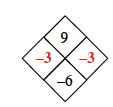### Home > CAAC > Chapter 1 > Lesson 1.2.2 > Problem1-57

1-57.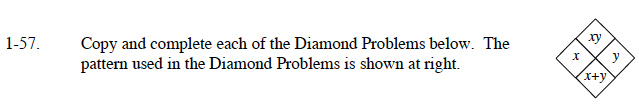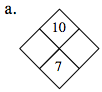What are the factors of 10?

1 and 10
2 and 5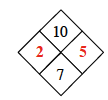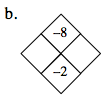Find the factors of −8.

The larger factor will be the negative one.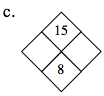Follow the same steps taken in (a).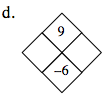Remember that when two negative numbers are multiplied the answer is positive, and when they are added together the answer is negative.